A revealing photograph

While looking around on Flickr for images for the previous post, I came across this captivating photograph taken by Toni Frissell.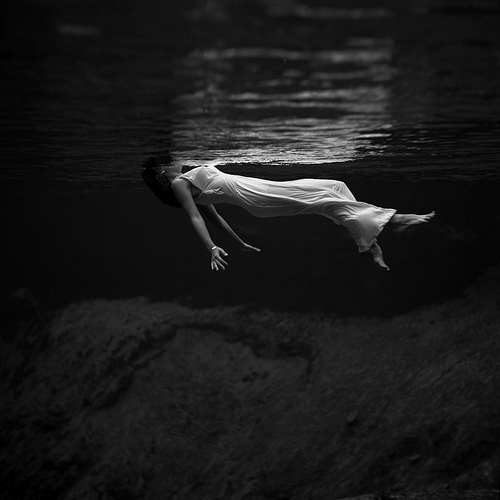More than meets the eye?

It’s a gorgeous shot on aesthetic grounds. Perfect lighting and composition, a beautiful subject, and a strikingly dramatic moment. And seen another way, it’s a metaphor for what Empirical Zeal is all about: diving beneath the surface, and looking at things from a different point of view.

It turns out that this photograph is a neat illustration of two interesting physical phenomena. Can you guess what they are? And here’s another (admittedly odd) question. Can we use this photograph to work out the density of this woman?

Well, the first principle that this photo demonstrates is the idea of buoyancy. Objects that are submerged in a fluid (in this case water) experience a force that helps them float. Why does this happen? Imagine that instead of the woman, you had a woman shaped hole in the water. The water would try to rush in and fill the cavity. So even when the woman is underwater, the water is constantly pushing against her.

Mostly, she doesn’t feel this because the pushes cancel out. The water is pushing her to the left just as much as it is to the right, and she moves neither left nor right. But here’s the kicker - the water under the woman is at a slightly higher pressure than the water above her (water pressure increases with depth). In other words, the water below her is pushing harder than the water above her. The net result is an upwards force provided by the water, and this is called the buoyant force.

If this buoyant force of the water matches her weight, then she will float. If her weight exceeds it, she will sink. That’s why rocks sink, and beach balls don’t.

But how can we workout her density? To do this we need to recall two things from high school physics.

1. The weight of an object is its mass multiplied by g = 9.8 N/kg

2. The buoyant force is equal to the weight of the fluid displaced by the object (in this case the weight of water that the lady has pushed out of her way)

And now we can start cooking. The first thing you do in any physics problem is draw a picture.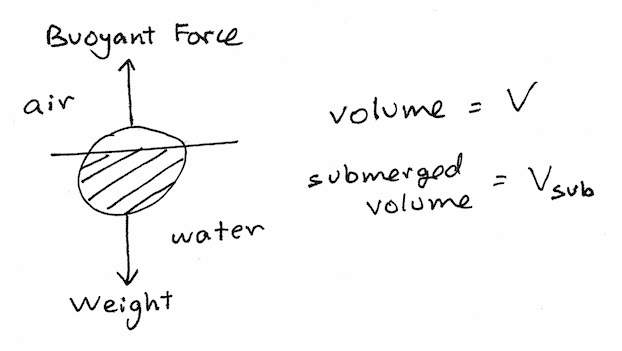Consider a spherical woman. Certainly not a flattering portrait, but fortunately the shape doesn’t matter at all.

We see that there are two force acting on the woman. The first force is her weight, pulling her down.

$latex \textrm{Weight} = m g$

We can then rewrite this as

$latex \textrm{Weight} = \rho V g$

where $latex \rho$ is her density, and $latex V$ is her volume.

The second force is the buoyant force, which is given by the weight of water that she displaces.

$latex \textrm{Buoyant force} = m_{sub} g$

where $latex m_{sub}$ is the mass of water displaced by the lady (the shaded region in the above figure)

Like before, we can write this in terms of density, so that

$latex \textrm{Buoyant force} = \rho_{water} V_{sub} g$

where $latex \rho_{water}$ is the density of water and $latex V_{sub}$ is the volume of the lady that is submerged in water.

Now, for the woman to remain stationary as she is in the photograph, the two forces must balance each other out:

$latex \textrm{Weight} = \textrm{Buoyant force}$

or $latex \rho V g = \rho_{water} V_{sub} g$

Rearranging this equation gives us the density of the lady:

$latex \rho= \rho_{water} (V_{sub}/V)$

Happily, the answer doesn’t depend on her shape. It also doesn’t depend on the value of gravitational acceleration $latex g$, which means that if you were to repeat this photo shoot on a lake on the moon, it would look essentially the same.

In words, this equation tells us that **the density of the lady is the density of water multiplied by the percentage of the lady that is submerged underwater. **

** **The photo was taken in a place called Weeki Wachi Springs in Florida, so this must be fresh water, whose density is 1 kilogram per liter. Looking at the picture, it seems like only half her head is above the water. Assuming that a head takes up 10% of the body’s volume, this means that 95% of the lady is submerged. So her density is 95% that of water, or 0.95 kilograms per liter.

If we wanted, we could also work out her mass. To do this we need to estimate her volume. I have no idea what this is, but it’s a number between 50 and 100 litres. Her mass is then her density (0.95 kg/L) multiplied by her volume.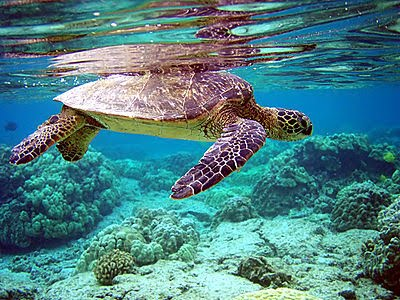And now, for something completely different..

The second physics principle in this photograph comes from the field of optics. If you look at the surface of the water, you can see that it behaves like a mirror, reflecting the light from her dress. Water is usually transparent, so why is it reflecting light?

What’s going on here is a phenomenon known as total internal reflection. This happens because light rays bend outwards as they go from water to air. Depending on the angle, some rays can make it out, while others are trapped and they bounce back.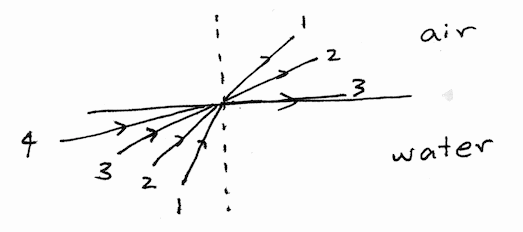Rays 1 and 2 can exit the water, ray 3 skims along the surface, and ray 4 is trapped and reflects back down instead.

You can see this happen if you stand underwater in clear water and look up. You’ll be able to see the sky inside a circle above your head, but outside that circle, the rest of the water surface becomes a mirror, reflecting whatever is under it.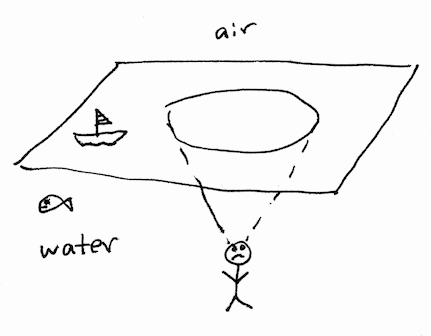Cone of loneliness. This young lad will see two fish, one that is reflected on the water surface.

And so, here we are. We’ve dissected a beautiful photograph and arrived at some grotesque caricatures. While they may not be much to look at, they represent  conceptual shifts in our understanding of the world. And we enrich our world by getting to know them better.